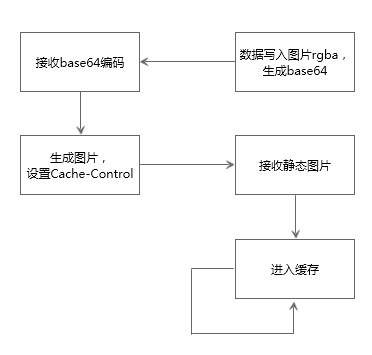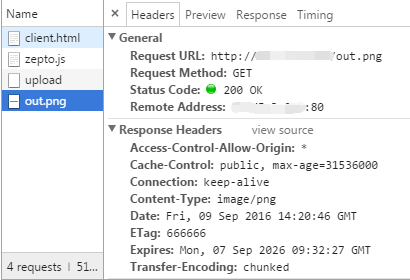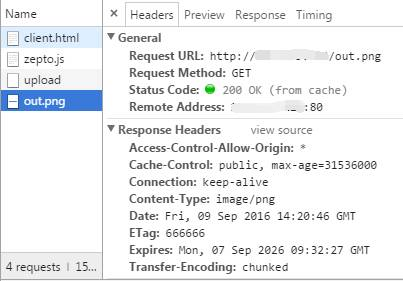const fs = require('fs')

const http = require('http')

const url = require('url')

const querystring = require('querystring')

const util = require('util')

const server = http.createServer((req, res) => {

let pathname = url.parse(req.url).pathname

let realPath = 'assets' + pathname

console.log(realPath)

if (realPath !== 'assets/upload') {

fs.readFile(realPath, "binary", function(err, file) {

if (err) {

res.writeHead(500, {'Content-Type''text/plain'})

res.end(err)

} else {

res.writeHead(200, {

'Access-Control-Allow-Origin''*',

'Content-Type''image/png',

'ETag'"666666",

'Cache-Control''public, max-age=31536000',

'Expires''Mon, 07 Sep 2026 09:32:27 GMT'

})

res.write(file, "binary")

res.end()

}

})

} else {

let post = ''

req.on('data', (chunk) => {

post += chunk

})

req.on('end', () => {

post = querystring.parse(post)

console.log(post.imgData)

res.writeHead(200, {

'Access-Control-Allow-Origin''*'

})

let base64Data = post.imgData.replace(/^data:image\/\w+;base64,/, "")

let dataBuffer = new Buffer(base64Data, 'base64')

fs.writeFile('assets/out.png', dataBuffer, (err) => {

if (err) {

res.write(err)

res.end()

}

res.write('OK')

res.end()

})

})

}

})

server.listen(80)

console.log('Listening on port: 80')

res.writeHead(200, {

'Access-Control-Allow-Origin''*',

'Content-Type''image/png',

'ETag'"666666",

'Cache-Control''public, max-age=31536000',

'Expires''Mon, 07 Sep 2026 09:32:27 GMT'

})

<!-- client.html -->

<canvas id="canvas" width="8", height="1"></canvas>

<!-- client.js -->

let keyString = '01234567890123456789012345678901'

let canvas = document.querySelector('#canvas')

let ctx = canvas.getContext('2d')

let imgData = ctx.createImageData(8, 1)

for (let i = 0; i < imgData.data.length; i += 4) {

imgData.data[i + 0] = parseInt(keyString[i]) + 50

imgData.data[i + 1] = parseInt(keyString[i + 1]) + 100

imgData.data[i + 2] = parseInt(keyString[i + 2]) + 150

imgData.data[i + 3] = parseInt(keyString[i + 3]) + 200

}

ctx.putImageData(imgData, 0, 0)

// console.log(imgData.data)

[50, 101, 152, 203, 54, 105, 156, 207, 58, 109, 150, 201, 52, 103, 154, 205, 56, 107, 158, 209, 50, 101, 152, 203, 54, 105, 156, 207, 58, 109, 150, 201]

\$.post('http://xx.xx.xx.xx:80/upload', { imgDatacanvas.toDataURL() }, (data) => {

if (data === 'OK') {

let img = new Image()

img.crossOrigin = "anonymous"

img.src = 'http://xx.xx.xx.xx:80/out.png'

img.onload = () => {

console.log('完成图片请求与缓存')

ctx.drawImage(img, 0, 0)

console.log(ctx.getImageData(0, 0, 8, 1).data)

}

}

})200 OK，证明是从服务端获取的图片。200 OK (from cache)，证明是从本地缓存读取的图片。